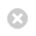Close

Mathematics

Home# Mathematical Physics

Gravitation and cosmology. Dynamical dark energy models. Mathematical foundations of general relativity and quantum theory,Kaluza-Klein theories and non-standard spinors. Alternative non-Riemannian field theories. Integrable systems in the form of differential, discrete and ultra discrete equations. Painleve equations and novel reductions of the self-dual Yang-Mills equations such as the Darboux-Halphen system.